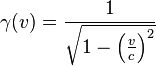LaTeX Power for HTML5 Web-Server

$latex \LaTeX&fg=880088&s=2$, an amazing clever document preparation system, which is super helpful to format absolut (100%) correctly, very complex mathematical formulas and equations for any professional document and educational books, can now also directly be used in modern CMS/Web-Systems – like WordPress. http://en.support.wordpress.com/latex

Simple examples of formulas written in $latex \LaTeX&fg=880088&s=1$:

$latex \boxed{ E = mc^2 }&bg=ffff00&s=3$

$latex \boxed{ E_k = \frac{mc^2}{\sqrt{1-(\frac{v}{c})^2}} }&fg=ff0000&s=3$

Lorentzfactor (designed with $latex \LaTeX&fg=0000ff&s=1$):   $latex \boxed{ \gamma(v) = \frac{1}{\sqrt{1-(\frac{v}{c})^2}} }&fg=0000ff&s=2$

vs. Lorentzfactor (inserted as a picture):$\gamma(v) = \frac 1 {\sqrt{1 - \left( \frac{v}{c} \right) ^2}}$

$latex \boxed{ M = \frac{m}{\sqrt{1-(\frac{v}{c})^2}} }&fg=884488&s=3$

$latex \sqrt{\frac{n}{n-1} S}&s=3$

$latex e^{i \pi} + 1 = 0&s=3$

$latex i\hbar\frac{\partial}{\partial t}\left|\Psi(t)\right>=H\left|\Psi(t)\right>&s=3$

$latex \begin{pmatrix} \alpha& \beta^{*}\\ \gamma^{*}& \delta \end{pmatrix}&s=3$

$latex \mathbf{X} = \left( \begin{array}{ccc} x_{11} & x_{12} & \ldots \\ x_{21} & x_{22} & \ldots \\ \vdots & \vdots & \ddots \end{array} \right)$

$latex 2^k-\binom{k}{1}2^{k-1}+\binom{k}{2}2^{k-2}&s=3$

$latex \boxed{\ ifs^3 \ge (AWS)^2 + (Microsoft\ Azure)^2 + (Google\ Cloud\ Platform)^2\ }&bg=666666&fg=ffffff&s=3$

The English short description for $latex \LaTeX&s=0$$latex 2\epsilon is: http://tug.ctan.org/info/lshort/english/lshort.pdf The German short description for latex \LaTeX&s=0$$latex 2\epsilon$ is:
http://tug.ctan.org/info/lshort/german/l2kurz.pdf

The official $latex \LaTeX&s=0$ – Website:  http://www.latex-project.org is also a helpful place to have a look into $latex \LaTeX&s=0$.
The German TeX User-Group is: http://www.dante.de
The German Wikipedia for $latex \LaTeX&s=0$ is: https://de.wikipedia.org/wiki/LaTeX